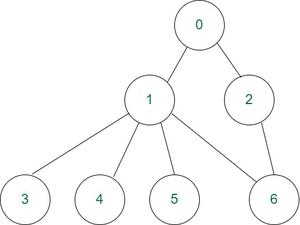GeeksforGeeks App
Open AppBrowser
Continue

# C program to implement DFS traversal using Adjacency Matrix in a given Graph

Given a undirected graph with V vertices and E edges. The task is to perform DFS traversal of the graph.

Examples:

Input: V = 7, E = 7
Connections: 0-1, 0-2, 1-3, 1-4, 1-5, 1-6, 6-2
See the diagram for connections:Output : 0 1 3 4 5 6 2
Explanation: The traversal starts from 0 and follows the following path 0-1, 1-3, 1-4, 1-5, 1-6, 6-2.

Input: V = 1, E = 0
Output: 0
Explanation: There is no other vertex than 0 itself.

Approach: Follow the approach mentioned below.

• Initially all vertices are marked unvisited (false).
• The DFS algorithm starts at a vertex u in the graph. By starting at vertex u it considers the edges from u to other vertices.
• If the edge leads to an already visited vertex, then backtrack to current vertex u.
• If an edge leads to an unvisited vertex, then go to that vertex and start processing from that vertex. That means the new vertex becomes the current root for traversal.
• Follow this process until a vertices are marked visited.

Here adjacency matrix is used to store the connection between the vertices.

Take the following graph:The adjacency matrix for this graph is:

Below is implementations of simple Depth First Traversal.

## C

 `// C code to implement above approach``#include ``#include ` `// Globally declared visited array``int` `vis;` `// Graph structure to store number``// of vertices and edges and``// Adjacency matrix``struct` `Graph {``    ``int` `V;``    ``int` `E;``    ``int``** Adj;``};` `// Function to input data of graph``struct` `Graph* adjMatrix()``{``    ``struct` `Graph* G = (``struct` `Graph*)``        ``malloc``(``sizeof``(``struct` `Graph));``    ``if` `(!G) {``        ``printf``(``"Memory Error\n"``);``        ``return` `NULL;``    ``}``    ``G->V = 7;``    ``G->E = 7;` `    ``G->Adj = (``int``**)``malloc``((G->V) * ``sizeof``(``int``*));``    ``for` `(``int` `k = 0; k < G->V; k++) {``        ``G->Adj[k] = (``int``*)``malloc``((G->V) * ``sizeof``(``int``));``    ``}` `    ``for` `(``int` `u = 0; u < G->V; u++) {``        ``for` `(``int` `v = 0; v < G->V; v++) {``            ``G->Adj[u][v] = 0;``        ``}``    ``}``    ``G->Adj = G->Adj = 1;``    ``G->Adj = G->Adj = 1;``    ``G->Adj = G->Adj = 1;``    ``G->Adj = G->Adj = 1;``    ``G->Adj = G->Adj = 1;``    ``G->Adj = G->Adj = 1;``    ``G->Adj = G->Adj = 1;` `    ``return` `G;``}` `// DFS function to print DFS traversal of graph``void` `DFS(``struct` `Graph* G, ``int` `u)``{``    ``vis[u] = 1;``    ``printf``(``"%d "``, u);``    ``for` `(``int` `v = 0; v < G->V; v++) {``        ``if` `(!vis[v] && G->Adj[u][v]) {``            ``DFS(G, v);``        ``}``    ``}``}` `// Function for DFS traversal``void` `DFStraversal(``struct` `Graph* G)``{``    ``for` `(``int` `i = 0; i < 100; i++) {``        ``vis[i] = 0;``    ``}``    ``for` `(``int` `i = 0; i < G->V; i++) {``        ``if` `(!vis[i]) {``            ``DFS(G, i);``        ``}``    ``}``}` `// Driver code``void` `main()``{``    ``struct` `Graph* G;``    ``G = adjMatrix();``    ``DFStraversal(G);``}`

Output

`0 1 3 4 5 6 2 `

Time Complexity: O(V + E)
Auxiliary Space: O(V)

My Personal Notes arrow_drop_up